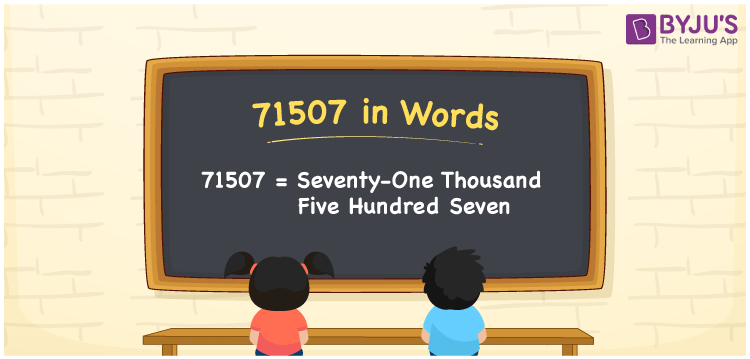# 71507 in words

71507 in words is written as Seventy One Thousand Five Hundred and Seven. In 71507, 7 has a place value of ten thousand, 1 has a place value of thousand, 5 is in the place value of hundred, and 7 is in the place value of one. The article on Place Value gives more information. The number 71507 is used in expressions that relate to money, distance, length, social media views, and many more. For example, “A popular real estate brand has constructed Seventy One Thousand Five Hundred and Seven houses across the world.”

 71507 in words Seventy One Thousand Five Hundred and Seven Seventy One Thousand Five Hundred and Seven in Numbers 71507

## 71507 in English Words## How to Write 71507 in Words?

We can convert 71507 to words using a place value chart. This can be done as follows. The number 71507 has 5 digits, so let’s make a chart that shows the place value up to 5 digits.

 Ten thousand Thousands Hundreds Tens Ones 7 1 5 0 7

Thus, we can write the expanded form as:

7 × Ten thousand + 1 × Thousand + 5 × Hundred + 0 × Ten + 7 × One

= 7 × 10000 + 1 × 1000 + 5 × 100 + 0 × 10 + 7 × 1

= 71507.

= Seventy One Thousand Five Hundred and Seven.

71507 is the natural number that is succeeded by 71506 and preceded by 71508.

71507 in words – Seventy One Thousand Five Hundred and Seven.

Is 71507 an odd number? – Yes.

Is 71507 an even number? – No.

Is 71507 a perfect square number? – No.

Is 71507 a perfect cube number? – No.

Is 71507 a prime number? – No.

Is 71507 a composite number? – Yes.

## Solved Example

1. Write the number 71507 in expanded form

Solution: 7 x 10000 + 1 x 1000 + 5 x 100 + 0 x 10 + 7 x 1

We can write 71507 = 70000 + 1000 + 500 + 00 + 7

= 7 x 10000 + 1 x 1000 + 5 x 100 + 0 x 10 + 7 x 1.

## Frequently Asked Questions on 71507 in words

Q1

### How to write the number 71507 in words?

71507 in words is written as Seventy One Thousand Five Hundred and Seven.
Q2

### Is 71507 divisible by 2?

No. 71507 is not divisible by 2.
Q3

### Is 71507 a prime number?

No. 71507 is not a prime number.